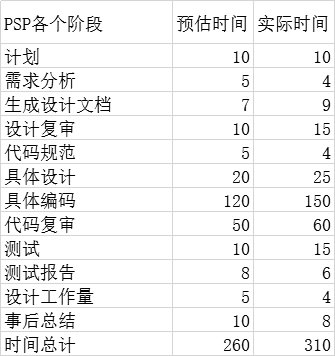# 1. 需求分析：

为了对学生的算数进行锻炼，家长每天给孩子出题成为一个难题，需要做出一个程序，每天生成一定数量且不重复的题目，分解家长们的压力。

# 2. 功能设计：

基本功能：

1. 自动生成10道100以内的2个操作数的四则运算算式（+ - *  /），要求运算结果也在100以内 ------>实现
2. 剔除重复算式。  2 + 3 =    和  2 + 3 =     是重复算式      2 + 3 =   和   3 + 2 =  不属于重复算式 ------>实现
3. 题目数量可定制 ------>实现
4. 相关参数可控 ------>实现
1.  是否包含乘法和除法

2. 操作数数值范围可控（如操作数 在100以内   还是1000以内）

3. 操作数是否含负数

5. 生成的运算题存储到外部文件result.txt中 ------->实现

拓展功能：

自动生成答案

添加学号，并为每个学号生成不同的试题

# 3. 设计实现：

1. 使用构造函数PrintStream Scanner ，PrintStream ps = new PrintStream("d:/result.txt");
2. System.setOut(ps);调用此函数，可以将所有System.out.println();输出语句，打印到i我们创建的文件夹中
3. Scanner t=new Scanner(System.in); 控制我们从键盘输入
4. Math.random();产生随机不重复函数，我做了以下测试
``` 1 /*
2  * 验证random会不会产生相同的随机数
3  * 做个10万次循环看看，发现结果为0
4  *java不像c,c会大概率产生相同的随机数，甚至会下次运行可能会和上次
5  * 产生的随机数是一样的，因此用C写得初始化一个时间种子，才能尽可能的保证随机数不相同
6  *java的random函数10万次运行都很难出现一次相同的随机数，所有random不产生重复函数
7  */
8 public class text_002{
9     public static void main(String args[]){
10             int count = 0;
11             double first = 0;
12             double second = 0;
13             for(int i=0; i<99999; i++){
14                 first = Math.random();
15                 second = Math.random();
16                 if (first == second)
17                     count ++;
18                 }
19             System.out.print(count);
20     }
21 }```

# 4. 测试运行：

基本加减法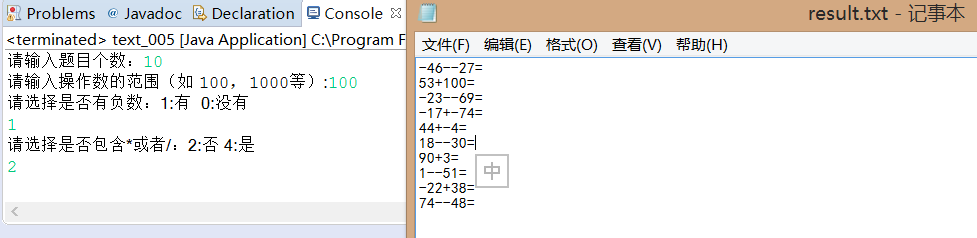四种运算符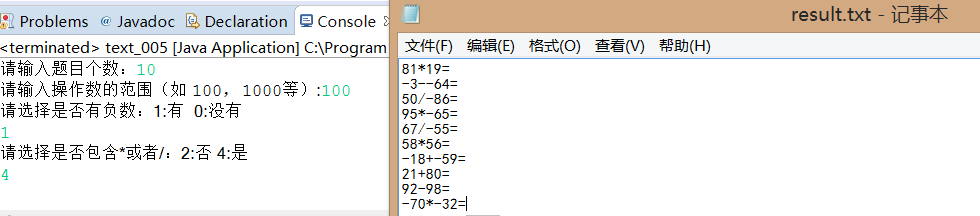自动生成答案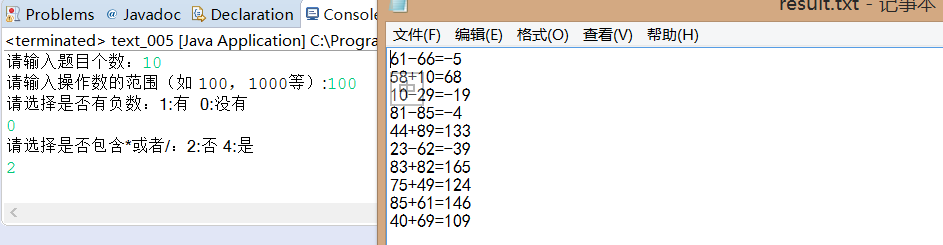四个运算符生成答案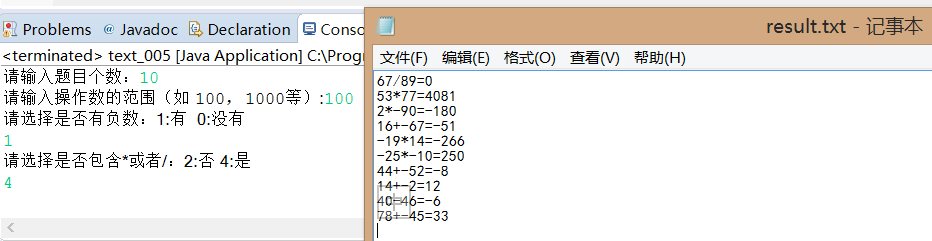添加学号，并且给不同部分学号的生产不同的套题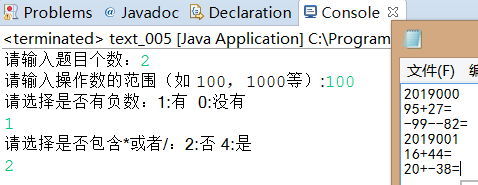# 5. 代码解释

• 　　生成的第一个操作数
``` 1 //第一个操作数的选择
2             int a=(int)(Math.random()*tf+1);
3
4             if(zf==1) {//有负数
5             int p=(int) (Math.random()*2);
6                 switch(p) {
7                 case 0:a=a*(-1);//取负数
8                        System.out.print(a);break;
9                 case 1:
10                           System.out.print(a);break;
11                 }
12             }
13             if(zf==0) {//没有负数
14                     System.out.print(a);
15                     }```
• 　　运算符号的选择
``` 1 System.out.println("请选择是否包含*或者/：2:否 4:是 ");
2         int fu=t.nextInt();
3 if(fu==2) {//选择两个字符‘+’‘-’
4                 int k=(int)(Math.random()*2);
5                 switch(k) {//随机选择运算符
6                 case 0:System.out.print("+");
7                        break;
8                 case 1:System.out.print("-");
9                        break;
10                                                }
11 if(fu==4) {//四个字符的‘+’‘-’‘*’‘/’
12             int k=(int)(Math.random()*4+1);
13             switch(k) {//随机选择运算符
14             case 1:System.out.print("+");break;
15             case 2:System.out.print("-");break;
16             case 3:System.out.print("*");break;
17             case 4:System.out.print("/");break;
18             }```
• 　　等号右侧结果
``` 1 //等号
2
3             System.out.print("=");
4             //运算结果
5             if(k==1) {
6                 c=a+b;System.out.println(c);
7             }
8             if(k==2) {
9                 c=a-b;System.out.println(c);
10             }
11             if(k==3) {
12                 c=a*b;System.out.println(c);
13             }
14             if(k==4) {
15                 c=a/b;System.out.println(c);
16             }```

# 6. 总结

 1 .控制题目个数 ：for循环 2. 控制题目范围 :scanner传参 和随机函数相乘 3. 控制正负号：随机函数+switch产生随机正负数 4. 控制运算符号：随机函数+switch产生随机符号

# 7. PSP